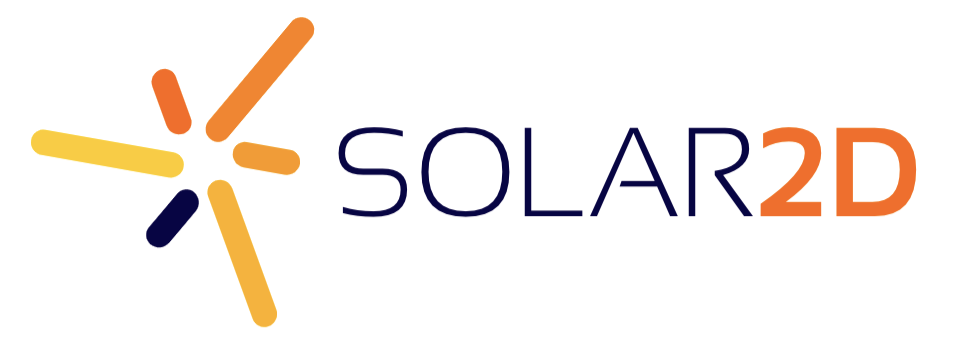# What should be the background images size to use with "ultimate" config file?

So I decided to use the “Tutorial: Modernizing the config.lua” located at http://coronalabs.com/blog/2013/09/10/modernizing-the-config-lua/

the code they provided is this one

``````--calculate the aspect ratio of the device: local aspectRatio = display.pixelHeight / display.pixelWidth application = { content = { width = aspectRatio \> 1.5 and 320 or math.ceil( 480 / aspectRatio ), height = aspectRatio \< 1.5 and 480 or math.ceil( 320 \* aspectRatio ), scale = "letterBox", fps = 30, imageSuffix = { ["@2x"] = 1.5, ["@4x"] = 3.0, }, }, }
``````

if I wanted to use this code in my config file then what sizes should my background images be? I want to make sure my backgrounds looks good on all devices, specifically on all iPhones. I will be making an app in portrait mode. Could someone please tell me the best resolution to use?

You should provide three background images:

360 x 570     – background.png

720 x 1140   – background@2x.png

1440 x 2280 – background@4x.png

Rob

Thank you Rob!

Hey Rob what about if i set the config.lua as

``````--calculate the aspect ratio of the device local aspectRatio = display.pixelHeight / display.pixelWidth application = { content = { width = aspectRatio \> 1.5 and 800 or math.ceil( 1200 / aspectRatio ), height = aspectRatio \< 1.5 and 1200 or math.ceil( 800 \* aspectRatio ), scale = "letterBox", fps = 30, imageSuffix = { ["@2x"] = 1.3, }, }, }
``````

then what would the background images be?

800 x 1200 is 2.5X as big as 320x480.  So multiply each number above by 2.5.  So the base image is 900 x 1425 and the @2x image would be 1800 x 2850.

Rob

You should provide three background images:

360 x 570     – background.png

720 x 1140   – background@2x.png

1440 x 2280 – background@4x.png

Rob

Thank you Rob!

Hey Rob what about if i set the config.lua as

``````--calculate the aspect ratio of the device local aspectRatio = display.pixelHeight / display.pixelWidth application = { content = { width = aspectRatio \> 1.5 and 800 or math.ceil( 1200 / aspectRatio ), height = aspectRatio \< 1.5 and 1200 or math.ceil( 800 \* aspectRatio ), scale = "letterBox", fps = 30, imageSuffix = { ["@2x"] = 1.3, }, }, }
``````

then what would the background images be?

800 x 1200 is 2.5X as big as 320x480.  So multiply each number above by 2.5.  So the base image is 900 x 1425 and the @2x image would be 1800 x 2850.

Rob